## 1.BCELoss

BCELoss又叫二分类交叉熵损失，顾名思义，它是用来做二分类的损失函数，我们先来看看BCELoss的公式。torch.manual_seed(0)
pt = torch.rand(2, 3)
target = torch.tensor([[0., 0., 1.], [1., 0., 0.]])
print(pt)
print(target)

pt我用的随机数代替的，target一般是0或者1，我们print一下，看看目前的数值是多少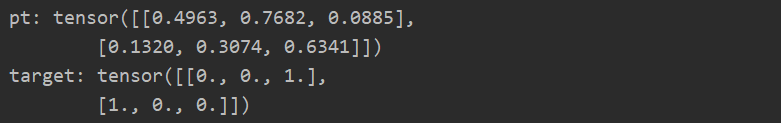pt的第一行第一列的值是 0.4963,它对应的标签target的第一行第一列的值是0，所以求根据刚才的公式L(pt,target) = -w*(target * ln(pt) + (1-target) * ln(1-pt))，w一般取1

L = -1 * (0*ln(0.4963)+1*ln(1-0.4963)) = -ln(1-0.4963) = 0.685774426230532 ≈ 0.6857

pt的第一行第二列的值是 0.7682,它对应的标签target的第一行第一列的值是0

L = -1 * (0*ln(0.7682)+1*ln(1-0.7682)) = -ln(1-0.7682) = 1.46188034807648  ≈ 1.4620

pt的第一行第三列的值是 0.0885,它对应的标签target的第一行第一列的值是1

L = -1 * (1*ln(0.0885)+0*ln(1-0.0885)) = -ln(0.0885) = -2.424752726968253  ≈ 2.4250

    def com(x, y):
loss = -(y * torch.log(x) + (1 - y) * torch.log(1 - x))
return loss

losss = com(pt, target)
print(losss)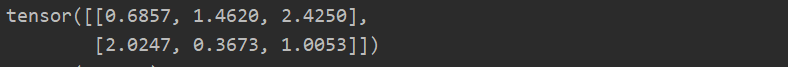所以loss = （1.524233333333333333333 + 1.132433333333333333333）/ 2 ≈1.328333

上代码看看是不是这么回事吧

    torch.manual_seed(0)
pt = torch.rand(2, 3)
target = torch.tensor([[0., 0., 1.], [1., 0., 0.]])
print('pt:',pt)
print('target:',target)

def com(x, y):
loss = -(y * torch.log(x) + (1 - y) * torch.log(1 - x))
return loss
losss = com(pt, target)
print(losss)
losss = torch.mean(com(pt, target))
print('总loss：',losss)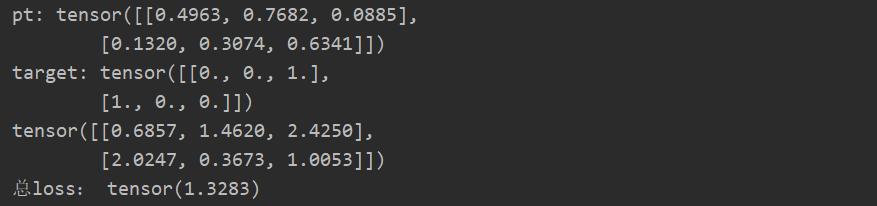不错，一模一样，算对了。但是你肯定有疑问了，你这是你自己手算的，代码也是你自己写的，你只能证明你的计算和你的代码是对上了，怎么证明真正的和BCELoss对上了，那我们请出Pytorch的nn.BCELoss来看看结果吧

torch.manual_seed(0)
pt = torch.rand(2, 3)
target = torch.tensor([[0., 0., 1.], [1., 0., 0.]])
print('pt:',pt)
print('target:',target)
loss = nn.BCELoss()
print('pytorch loss:',loss(pt, target))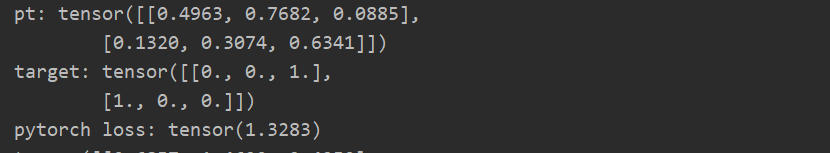## 2.带权重的BCELosstorch.nn.BCELoss()中，其实提供了一个weight的参数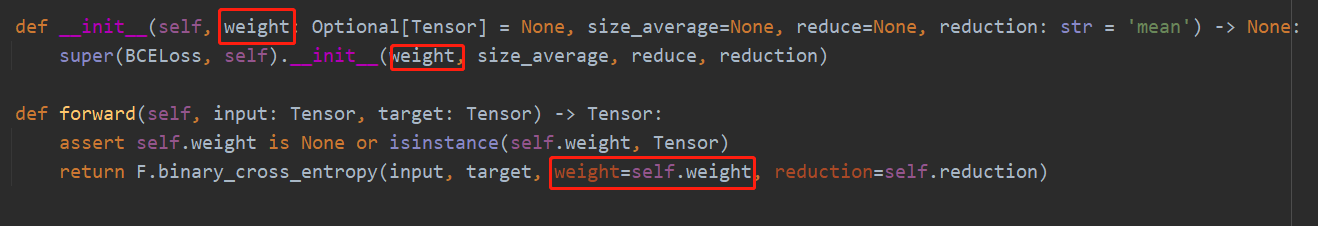class BCE_WITH_WEIGHT(torch.nn.Module):
def __init__(self, alpha=0.25, reduction='mean'):
super(BCE_WITH_WEIGHT, self).__init__()
self.alpha = alpha
self.reduction = reduction

def forward(self, predict, target):
pt = predict
loss = -((1-self.alpha) * target * torch.log(pt+1e-5) + self.alpha * (1 - target) * torch.log(1 - pt+1e-5))
if self.reduction == 'mean':
loss = torch.mean(loss)
elif self.reduction == 'sum':
loss = torch.sum(loss)

return loss

核心带代码是

loss = -((1-self.alpha) * target * torch.log(pt+1e-5) + self.alpha * (1 - target) * torch.log(1 - pt+1e-5))

alpha就是权重了，一般很多时候，正负样本是不平衡的，如果不加入权重，网络训练的时候，训练的关注的重点就跑到了样本多的那一类样本上去，对样本少的就不公平了，所以为了维护世界和平，贯彻爱与真实的邪恶，可爱又迷人的反派角色，带权重的损失函数就出现了。

## 3.BCE版本的Focal_Loss

FocalLoss的公式class BCEFocalLoss(torch.nn.Module):
def __init__(self, gamma=2, alpha=0.25, reduction='mean'):
super(BCEFocalLoss, self).__init__()
self.gamma = gamma
self.alpha = alpha
self.reduction = reduction

def forward(self, predict, target):
pt = predict
loss = - ((1 - self.alpha) * ((1 - pt+1e-5) ** self.gamma) * (target * torch.log(pt+1e-5)) +  self.alpha * (
(pt++1e-5) ** self.gamma) * ((1 - target) * torch.log(1 - pt+1e-5)))

if self.reduction == 'mean':
loss = torch.mean(loss)
elif self.reduction == 'sum':
loss = torch.sum(loss)
return loss

loss = - ((1 - self.alpha) * ((1 - pt+1e-5) ** self.gamma) * (target * torch.log(pt+1e-5)) +  self.alpha * (
(pt+1e-5) ** self.gamma) * ((1 - target) * torch.log(1 - pt+1e-5)))

Focalloss的目前不仅是为了控制样本不平衡的现象，还有个作用就是，让网络着重训练难样本。